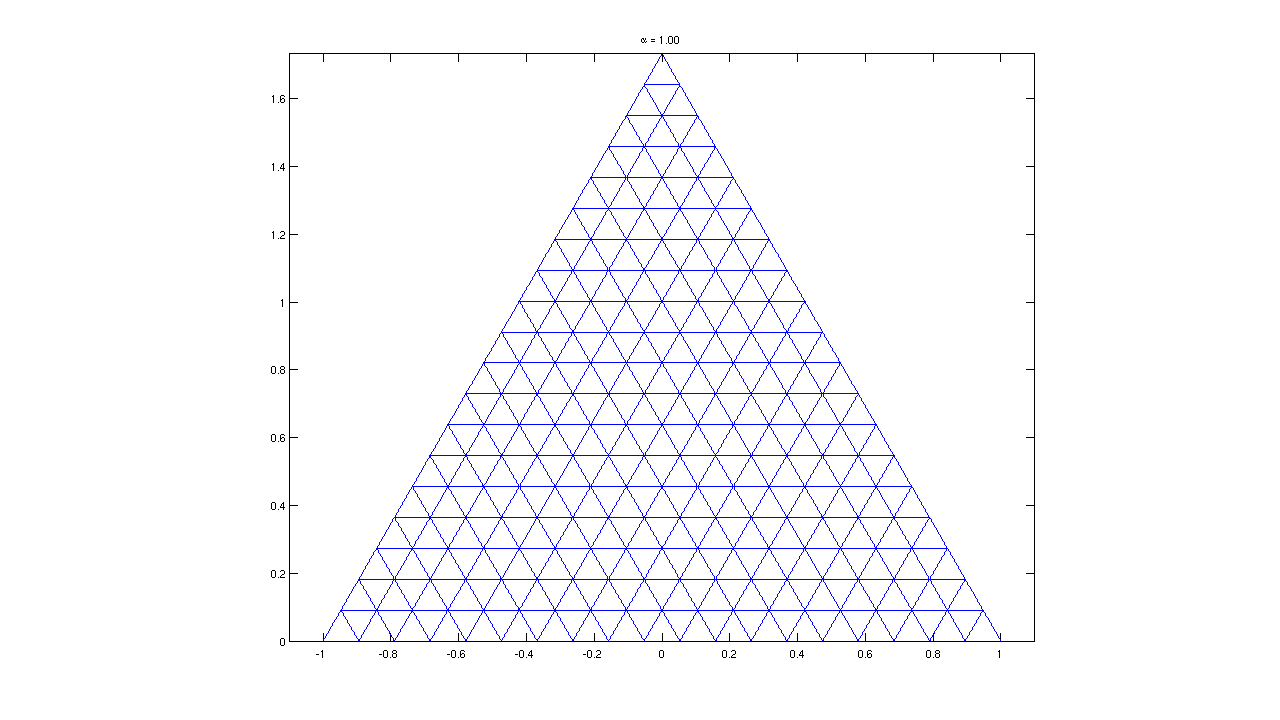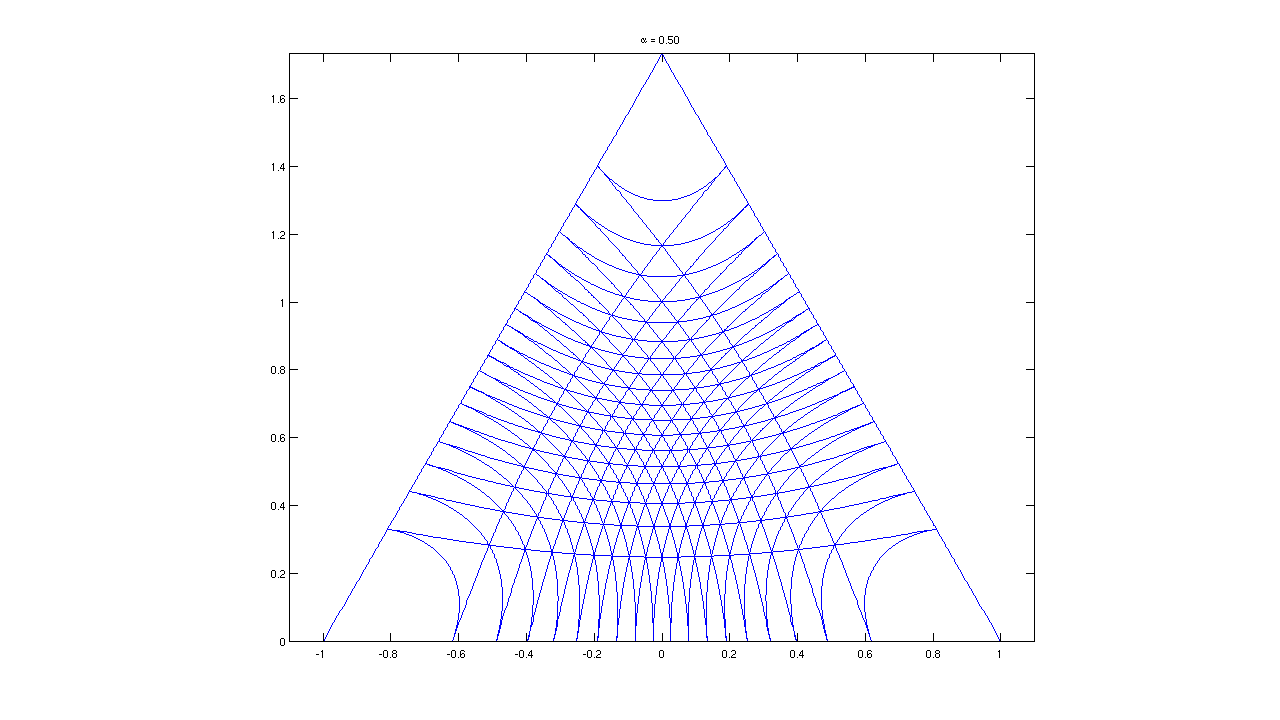# Visualising The Escort Probability Map

2016-08-11

Abstract

A simple visualisation of the escort probability map.

# Introduction

Fix a number $$\alpha > 0$$. We look at the mapping that takes a discrete distribution $$P$$ and outputs a new distribution $$Q$$ defined by $Q(a) ~=~ \frac{P(a)^\alpha}{\sum_a P(a)^\alpha} .$ This mapping is sometimes called the escort probability map (I do not know yet why). It is a continuous bijection from the simplex to itself. It maps the uniform distribution to itself, and corners to corners. For $$\alpha=1$$ it is the identity mapping.

To get a feeling for the effect of $$\alpha$$, let us put a regular triangular mesh on the 3 simplex. We can then graph the mesh after applying the escort probability map. For $$\alpha=1$$ we recover the input mesh:$$\alpha = 1$$: Identity mapping

Now let’s see what happens for $$\alpha > 1$$. We see that distributions are pushed towards the corners.$$\alpha = 1.5$$

and similarly for $$\alpha < 1$$ distributions are sucked toward the middle$$\alpha = 0.5$$

Here is a video interpolating smoothly from $$\alpha=0$$ to $$\alpha=2$$.